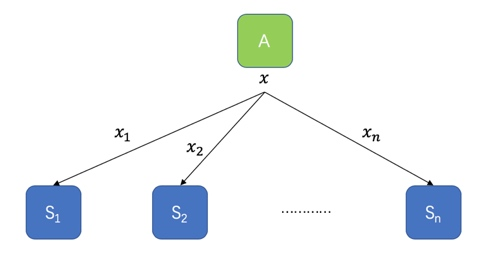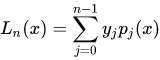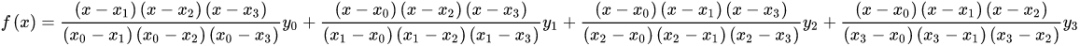## 密钥分享概念## 密钥分享实现

$a(x) =a_0+a_1x+a2x^2+...+a{m-1}x^{m-1}$

### 密钥恢复(recover)：

m个人把各自的密钥碎片聚合在一起，即m个点代入多项式函数，可以确定一条唯一的曲线，即计算出${a_0,a_1,a2,...,a{m-1}}$的值，其中的就是要恢复的秘密。

### 拉格朗日插值法

#### 使用方法：

$P_k(x)$是n-1次多项式，且满足$\forall m \in B_k$ 并且$P_k(m)=0, P_k(x_k)= 1$ 。最后得:n = 3 ,去掉式子中最后一个即可。

## 小结

### 相关阅读：

Schorr签名与椭圆曲线 Schorr签名与椭圆曲线

• 发表于 2020-11-20 14:30
• 阅读 ( 599 )
• 学分 ( 16 )
• 分类：入门/理论